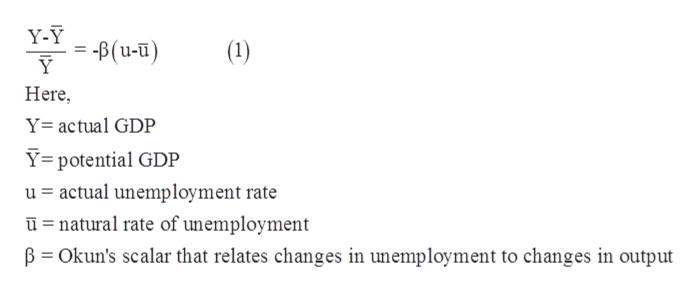# Suppose the unemployment rate is 9%, the natural rate of unemployment is 4.5%, and Okun’s scalar has an absolute value of .015. If potential GDP is $20T, what do you expect the actual GDP to be? (Round to nearest$10B)

Question
228 views

Suppose the unemployment rate is 9%, the natural rate of unemployment is 4.5%, and Okun’s scalar has an absolute value of .015. If potential GDP is $20T, what do you expect the actual GDP to be? (Round to nearest$10B)

check_circle

Step 1

Okun’s law measures the relationship between output and unemployment. According to Okun’s law for every 1% increase in unemployment the output gap increases by some percentage point.

Step 2

Mathematically it can be expressed as follows,help_outlineImage TranscriptioncloseY-Y = -B(u-u) Y (1) Here Y= actual GDP Y= potential GDP u actual unemployment rate u natural rate of unemployment B Okun's scalar that relates changes in unemployment to changes in output fullscreen
Step 3

Given informa...

### Want to see the full answer?

See Solution

#### Want to see this answer and more?

Solutions are written by subject experts who are available 24/7. Questions are typically answered within 1 hour.*

See Solution
*Response times may vary by subject and question.
Tagged in